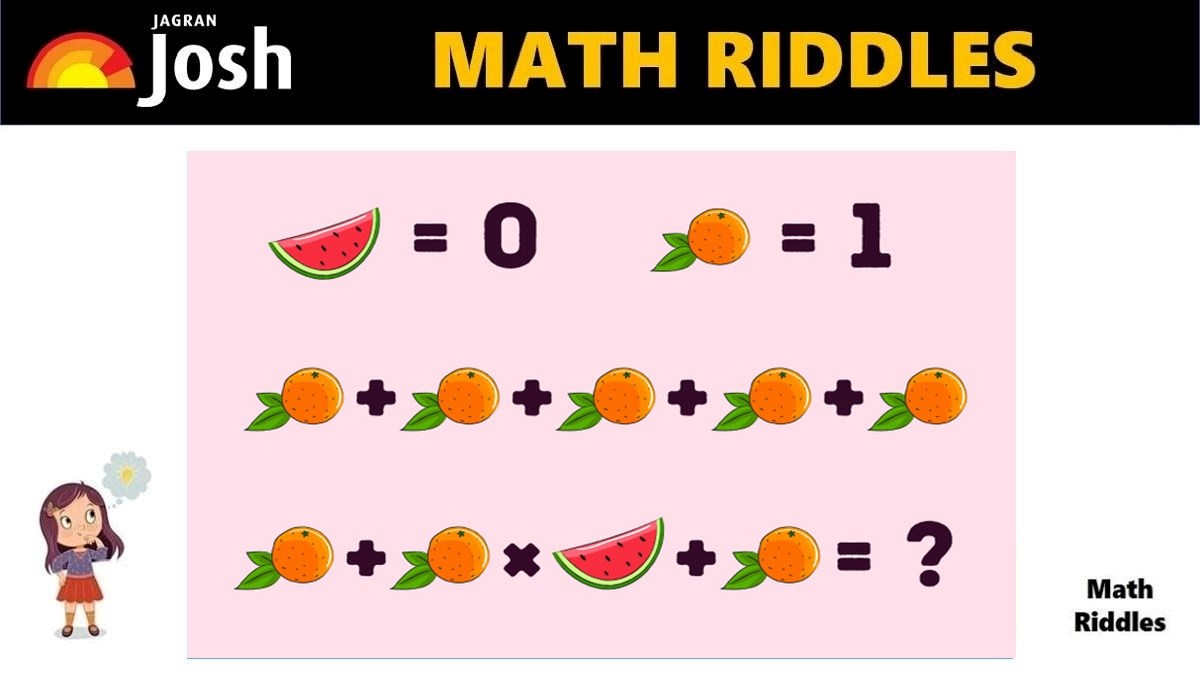# Math Riddles With Answers: Can You Solve This Tricky Math Rebus Puzzle 20 Seconds?

Rebus puzzles are a kind of brain teaser which can very cleverly hide a numerical expression. Are you a math genius? Only 1% with a high IQ can solve this tricky Rebus math puzzle in 20 seconds.Try to solve this Rebus Math Puzzle. Math enthusiasts who love solving tricky math puzzles and arithmetic problems are a big fan of Rebus Puzzles. Rebus Puzzles are pictogram puzzles that make your brain think harder and faster. You need a high level of logical reasoning and mathematical knowledge.

Rebus Puzzles are different from regular math puzzles. Why? Rebus puzzles are a kind of brain teaser which can very cleverly hide a numerical expression. In rebus puzzles, the numbers or arithmetic operations are encrypted with the use of pictures and words.

If you love solving cryptic rebus puzzles, give this math puzzle a try! You have 20 seconds to find the correct answer.

## Math Riddles with Answers: Solution

Explanation:

This is a Rebus Math Puzzle in which the arithmetic operations and numbers are encrypted with the help of pictures and words. They are presented in a mysterious confusing picture puzzle that can trick your brain in one go. One has to apply logical thinking to avoid getting tricked.

In this Math Riddle, if you just glance at the values given and apply them quickly to the equation, you will definitely make a mistake.

99% of people failed to find the right answer quickly within 20 seconds. They added up all the oranges in the first line with the equation in the second line. Some of them got 7 as their answer which is the incorrect answer.

Math Riddles With Answers: Can You Find The Value of Burger, Fries, Coke in 20 Seconds?

In this Rebus puzzle, there are actually two equations. However, the way they have been presented makes one think that they are connected with each other.

Look carefully at the picture, if all the oranges and watermelons were the same equation then there would a plus (+) sign either at the end of the first line of the equation or the beginning of the second line of the equation.

Therefore, the trick to solve this rebus puzzle is to add and multiply the values of the oranges and watermelon in the second line of the equation only.

Picture Puzzle: How Sharp Is Your Vision? Find Hidden Animals In The Picture In 11 Seconds

See the solution for the correct answer.

The value of watermelon is 0 and the value of orange is 1. We shall solve the second line of the equation only as there is no arithmetic operation or connector as explained above.

=> 1 + 1 x 0 + 1

=> 2

Hence, the correct answer is 2.

Great Work!

## Tell us in comments: Did you solve this rebus math puzzle correctly in 20 seconds?

Check out more amazing tricky math puzzles!

Math Riddles With Answers: Can You Find The Value of Each Candy in 20 Seconds?

Math Riddles With Answers: Can You Find The Value of Each Fruit in 20 Seconds?

Picture Puzzle: Find Two Same Emojis In 11 Seconds, 99% People Failed To Find Them

Picture Puzzle: Only 1% Genius Can Find The Hidden Words In The Picture in 11 seconds

Picture Puzzle: Find the Mistake In 11 Seconds, High Observational Skills Required

Get the latest General Knowledge and Current Affairs from all over India and world for all competitive exams.
खेलें हर किस्म के रोमांच से भरपूर गेम्स सिर्फ़ जागरण प्ले पर